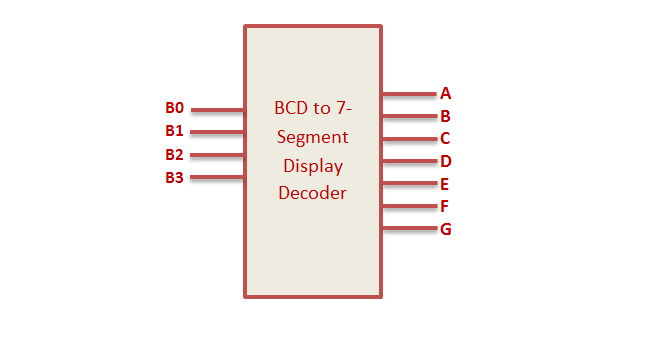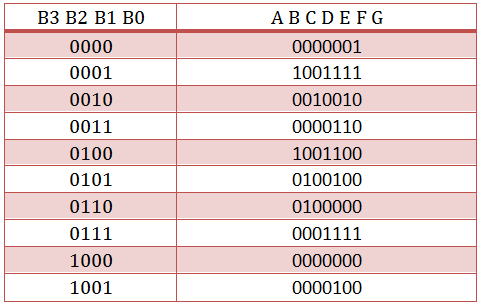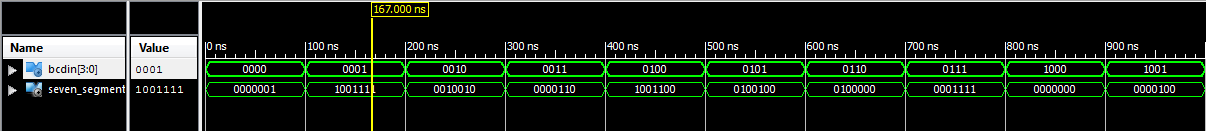# BCD to 7 Segment Decoder VHDL Code

### BCD to 7 Segment Decoder

The BCD to 7 Segment Decoder converts 4 bit binary to 7 bit control signal which can be displayed on 7 segment display. Seven display consist of 7 led segments to display 0 to 9 and A to F.VHDL Code BCD to 7 Segment Display decoder can be implemented in 2 ways. By simplifying Boolean expression to implement structural design and behavioral design.

For constructing BCD to 7 segment display, first construct truth table and simplify them to Boolean expression using K Map and finally build the combinational circuit.

### BCD to 7 segment display Decoder Truth Table### BCD to 7 segment display Decoder CircuitThe boolean expression for the logic circuit is

A = B0 + B2 + B1B3 + B1’B3′

B = B1′ + B2’B3′ + B2B3

C = B1 + B2′ + B3

D = B1’B3′ + B2B3′ + B1B2’B3 + B1’B2 + B0

E = B1’B3′ + B2B3′

F = B0 + B2’B3′ + B1B2′ + B1B3′

G = B0 + B1B2′ + B1’B2 + b2b3′

### VHDL Code for BCD to 7 segment display using Combinatorial logic

```
library IEEE;
use IEEE.STD_LOGIC_1164.ALL;

entity bcd_7seg is
Port ( B0,B1,B2,B3 : in STD_LOGIC;
A,B,C,D,E,F,G : out STD_LOGIC);
end bcd_7seg;

architecture Behavioral of bcd_7seg is

begin

A <= B0 OR B2 OR (B1 AND B3) OR (NOT B1 AND NOT B3);
B <= (NOT B1) OR (NOT B2 AND NOT B3) OR (B2 AND B3);
C <= B1 OR NOT B2 OR B3;
D <= (NOT B1 AND NOT B3) OR (B2 AND NOT B3) OR (B1 AND NOT B2 AND B3) OR (NOT B1 AND B2) OR B0;
E <= (NOT B1 AND NOT B3) OR (B2 AND NOT B3);
F <= B0 OR (NOT B2 AND NOT B3) OR (B1 AND NOT B2) OR (B1 AND NOT B3);
G <= B0 OR (B1 AND NOT B2) OR ( NOT B1 AND B2) OR (B2 AND NOT B3);

end Behavioral;

```

### VHDL Code for BCD to 7 segment display using Case Statement

```
library IEEE;
use IEEE.STD_LOGIC_1164.ALL;

entity bcd_7segment is
Port ( BCDin : in STD_LOGIC_VECTOR (3 downto 0);
Seven_Segment : out STD_LOGIC_VECTOR (6 downto 0));
end bcd_7segment;

architecture Behavioral of bcd_7segment is

begin

process(BCDin)
begin

case BCDin is
when "0000" =>
Seven_Segment <= "0000001"; ---0
when "0001" =>
Seven_Segment <= "1001111"; ---1
when "0010" =>
Seven_Segment <= "0010010"; ---2
when "0011" =>
Seven_Segment <= "0000110"; ---3
when "0100" =>
Seven_Segment <= "1001100"; ---4
when "0101" =>
Seven_Segment <= "0100100"; ---5
when "0110" =>
Seven_Segment <= "0100000"; ---6
when "0111" =>
Seven_Segment <= "0001111"; ---7
when "1000" =>
Seven_Segment <= "0000000"; ---8
when "1001" =>
Seven_Segment <= "0000100"; ---9
when others =>
Seven_Segment <= "1111111"; ---null
end case;

end process;

end Behavioral;

```

### VHDL Testbench Code for BCD to 7 segment display

```
LIBRARY ieee;
USE ieee.std_logic_1164.ALL;

ENTITY tb_bcd_7seg IS
END tb_bcd_7seg;

ARCHITECTURE behavior OF tb_bcd_7seg IS

-- Component Declaration for the Unit Under Test (UUT)

COMPONENT bcd_7segment
PORT(
BCDin : IN std_logic_vector(3 downto 0);
Seven_Segment : OUT std_logic_vector(6 downto 0)
);
END COMPONENT;

--Inputs
signal BCDin : std_logic_vector(3 downto 0) := (others => '0');

--Outputs
signal Seven_Segment : std_logic_vector(6 downto 0);

BEGIN

-- Instantiate the Unit Under Test (UUT)
uut: bcd_7segment PORT MAP (
BCDin => BCDin,
Seven_Segment => Seven_Segment
);

-- Stimulus process
stim_proc: process
begin

BCDin <= "0000";
wait for 100 ns;
BCDin <= "0001";
wait for 100 ns;
BCDin <= "0010";
wait for 100 ns;
BCDin <= "0011";
wait for 100 ns;
BCDin <= "0100";
wait for 100 ns;
BCDin <= "0101";
wait for 100 ns;
BCDin <= "0110";
wait for 100 ns;
BCDin <= "0111";
wait for 100 ns;
BCDin <= "1000";
wait for 100 ns;
BCDin <= "1001";
wait for 100 ns;
end process;

END;

```

### Testbench waveform for BCD to 7 Segment Display Decoder### 1 thought on “BCD to 7 Segment Decoder VHDL Code”

1.According to the truth table of this 7 segment decoder, the BCD input “0” is encoded as a dash, because segment G is active. Please not that people assume high-active logic unless stated otherwise. So you should either correct your truth table and equations or state that you are using a low-active 7-segment display. Please note further, that the display itself it not low-active. The low-activeness is mostly caused by switching transistors to drive the 7-segment inputs. These transistors cause a polarity inversion.